# Geometry Questions for SSC CGL Tier 2 PDF

0
3538

## Geometry Questions for SSC CGL Tier 2 PDF

Download SSC CGL Tier 2 Geometry Questions PDF. Top 10 SSC CGL Tier 2 Geometry questions based on asked questions in previous exam papers very important for the SSC exam.

Question 1: If an obtuse-angled triangle ABC, is the obtuse angle and O is the orthocenter. If = 54$^{\circ}$ , then is

a) 108$^{\circ}$

b) 126$^{\circ}$

c) 136$^{\circ}$

d) 116$^{\circ}$

Question 2: BC is the centre of the circle with centre O. A is a point on major arc BC as shown in the above figure. What is the value of $\angle{BAC}+\angle{OBC}$ ?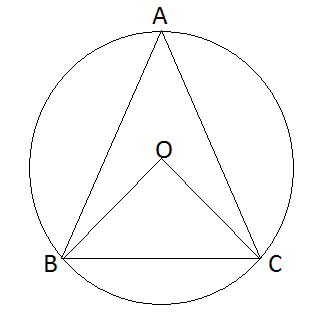a) 120$^{\circ}$

b) 60$^{\circ}$

c) 90$^{\circ}$

d) 180$^{\circ}$

Question 3: Internal bisectors of ∠Q and ∠R of ΔPQR intersect at O. If ∠ROQ = 96° then the value of ∠RPQ is

a) 36°

b) 24°

c) 12°

d) 6°

Question 4: In a ΔABC, ∠A+ ∠B = 118° ∠A + ∠C = 96°. find the value of ∠A.

a) 36°

b) 40°

c) 30°

d) 34°

Question 5: In a cyclic quadrilateral ∠A+∠C=∠B+∠D=?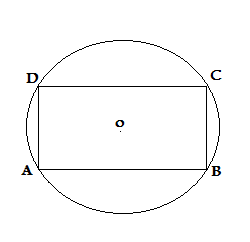a) 270°

b) 360°

c) 90°

d) 180°

Question 6: In the given figures, the lengths of the sides of ΔABC and ΔPQR are given and they are given in same units. Also ∠A and ∠B are given. Then value of ∠P is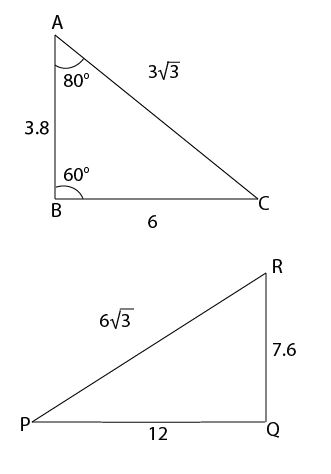a) 42°

b) 36°

c) 38°

d) 40°

Question 7: The area of a circle is proportional to the square of its radius. A small circle of radius 3 cm is drawn within a larger circle of radius 5 cm. Find the ratio of the areaof the annular zone to the area of the larger circle. (Area of the annular zone is the difference between the area of the larger circle and that of the smaller circle).

a) 9 : 16

b) 9 : 25

c) 16 : 25

d) 16 : 27

Question 8: In a triangle ABC, AB = AC, LBAC = 40° Then the external angle at B is :

a) 90°

b) 70°

c) 110°

d) 80°

Question 9: If the area of the circle in the figure is 36$\pi$ sq. cm. And ABCD is a square, then the area of $\triangle ACD$, in sq.cm, is

a) $12\pi$

b) $\frac{36}{\pi}$

c) 12

d) None of the above

Question 10: How many balls of radius 3 cm can be made by melting a bigger ball of diameter 48 cm?

a) 1024

b) 512

c) 64

d) 256

As we know that angle through an arc on centre is double that of made on remaining arc.
Hence $\angle{BOC}=2\angle{BAC}$=2x (where x=$\angle{BAC}$)
and  $\angle{OBC}$ would be 90-x
So  $\angle{BAC}+\angle{OBC}$=90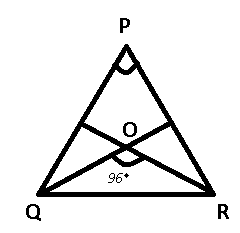To find : $\angle$RPQ = $\theta$ = ?

Solution : Let $\angle$PQR = $2x$ and $\angle$PRQ = $2y$

=> $\angle$OQR = $x$ and $\angle$ORQ = $y$ [SInce, QO & RO are angle bisectors]

In $\triangle$PQR

=> $\theta$ + $\angle$PQR + $\angle$PRQ = 180°

=> $\theta$ = 180° -2$(x+y)$ ———Eqn(1)

In $\triangle$QOR

=> x + y + 96° = 180°

=> x + y = 84°

Putting value of (x+y) in eqn (1)

=> $\theta$ = 180 – 2*84 = 180-168 = 12°

∠A + ∠B + ∠C = 180
∠A + ∠C = 96°,
∠B= 84, and
from ∠A+ ∠B = 118, ∠A= 34Sum of opposite angle of cyclic quadrilateral is 180° (D)

as we can see that in the given triangles

$\frac{AB}{RQ} = \frac{AC}{RP} = \frac{CB}{PQ} = \frac{1}{2}$

We know that if ratio of corresponding sides are equal in two triangles ,then the given traingles are similar and in similar triangles ass coressponding angles are also equal.

So , ∠A = ∠R , ∠C = ∠P , ∠B = ∠Q

C = 180 – ( 80 + 60) = 40

So ∠P = 40

Area of circle = $\pi r^2$

Area of annular zone = $\pi (5^2 – 3^2) = 16 \pi$ sq. units

Area of larger circle = $\pi * 5^2 = 25 \pi$ sq. units

=> Required ratio = 16 : 25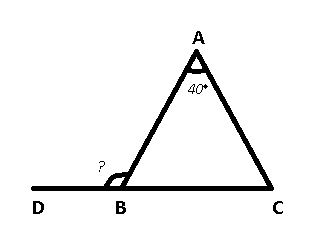Since AB = AC => $\angle$ACBC = $\angle$ACB

In $\triangle$ABC

=> $\angle$ABC + $\angle$ACB + $\angle$BAC = 180°

=> 2$\angle$ABC = 180° – 40° = 140°

=> $\angle$ABC = 70°

To find : $\angle$ABD = 180° – $\angle$ABC

= 180° – 70° = 110°

Area of circle = $\pi \times r^2= 36\pi$ sq.cm

=> $r = \sqrt{36}=6$ cm

=> Diameter of circle = $AC =2r = 12$ cm

In $\triangle$ ACD, let $AD=CD=s$ cm

=> $(AD)^2+(CD)^2=(AC)^2$

=> $2s^2=(12)^2=144$

=> $s^2=\frac{144}{2}=72$ ———–(i)

$\therefore$ Area of $\triangle$ ACD = $\frac{1}{2} \times s \times s$

= $\frac{s^2}{2}=36$ $cm^2$

=> Ans – (D)

Since the larger ball is melted, the volume will remain constant.

Radius of big ball = 24 cm and radius of small balls = 3 cm

Let $n$ balls be formed

=> $\frac{4}{3} \times \pi \times 3^3 \times n = \frac{4}{3} \times \pi \times 24^3$

=> $n = \frac{24^3}{3^3} = (\frac{24}{3})^3$

=> $n = 8^3 = 512$

=> Ans – (B)

We hope this Geometry Questions pdf for SSC CGL Tier 2 exam will be highly useful for your Preparation.